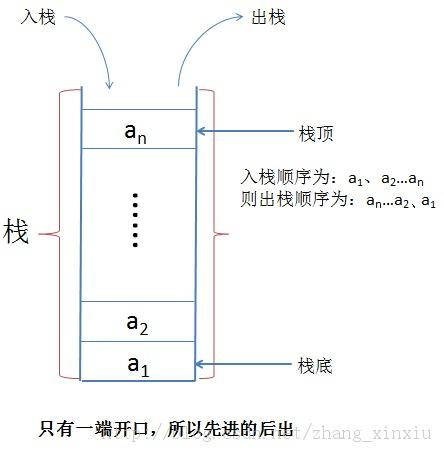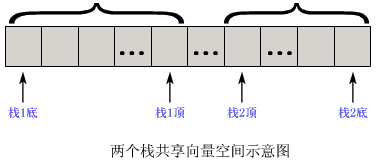（1） InitStack(S)

（2） ClearStack(S)

（3） IsEmpty(S)

（4） IsFull(S)

（5） Push(S,x)

（6） Pop(S, x)

（7） GetTop(S, x)

``````#define Stack_Size 50 typedef struct
{
StackElementType  elem[Stack_Size];   /*用来存放栈中元素的一维数组*/
int  top;  /*用来存放栈顶元素的下标，top 为-1 表示空栈*/
}SeqStack;
``````

``````//初始化
void  InitStack(SeqStack *S)
{/*构造一个空栈 S*/
S->top= -1;
}

//进栈
int Push(SeqStack * S, StackElementType x)
{
if(S->top== Stack_Size-1)
return(FALSE);  /*栈已满*/
S->top++;
S->elem[S->top]=x;
return(TRUE);
}

//出栈
int Pop(SeqStack * S, StackElementType *x)
{  /* 将栈 S 的栈顶元素弹出，放到 x 所指的存储空间中 */
if(S->top= =-1)  /*栈为空*/
return(FALSE);
else
{
*x= S->elem[S->top];
S->top--;/* 修改栈顶指针 */
return(TRUE);
}
}

//取栈顶元素。
int GetTop（SeqStack *S, StackElementType *x）
{  /* 将栈 S 的栈顶元素弹出，放到 x 所指的存储空间中，但栈顶指针保持不变 */       if(S->top==-1)  /*栈为空*/
return(FALSE);
else
{
*x = S->elem[S->top];
return(TRUE);
}
}
````````````//初始化操作。
void InitStack(DqStack *S)
{
S->top=-1;
S->top=M;
}

//进栈操作。
int Push(DqStack *S, StackElementType x, int i)
{/*把数据元素 x 压入 i 号堆栈*/
if(S->top+1==S->top) /*栈已满*/
return(FALSE);
switch(i)
{
case 0:
S->top++;
S->Stack[S->top]=x;
break;
case 1:
S->top--;
S->Stack[S->top]=x;
break;
default:  /*参数错误*/
return(FALSE)
}
return(TRUE);
}

//出栈操作。
int Pop(DqStack *S, StackElementType *x, int i)
{/* 从 i 号堆栈中弹出栈顶元素并送到 x 中 */
switch(i)
{
case 0:
if(S->top==-1)
return(FALSE);
*x=S->Stack[S->top];
S->top--;
break;
case 1:
if(S->top==M)
return(FALSE);
*x=S->Stack[S->top];
S->top++;
break;
default:
return(FALSE);
}
return(TRUE);
}
``````